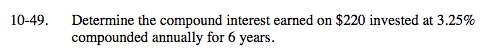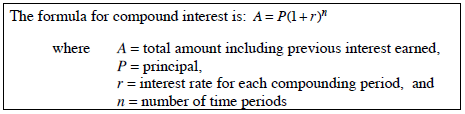### Home > MC2 > Chapter 10 > Lesson 10.1.4 > Problem10-49

10-49.According to the Math Notes box from Lesson 10.1.3:Using this formula, substitute the given values to solve for the total amount.

After solving for the total amount with compound interest, find the difference between the new total amount and the original principal to find the compound interest earned.

\$46.54